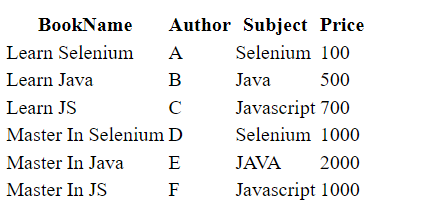GeeksforGeeks App
Open AppBrowser
Continue

# How to Handle Static Web Tables using Selenium WebDriver using Java?

Web Tables are used to organize similar types of content on the web page, Web tables are groups of elements that are logically stored in a row and column format. Web table has various HTML tags like., table, th, tr, td. Let’s understand these tags a bit more:

• <table> – It defines a table
• <th> – It defines a header cell
• <tr> – It defines a row in a table.
• <td>- It defines a cell in a table. the <td> tag always lies inside the <tr> tag.

### Example of HTML Table

Static Demo Table

The below table is a static demo table and the HTML code for the table:

## HTML

 `<``html``>``   ``<``head``>``      ``<``style``>``   ````   ``<``body``>``      ``<``table` `name``=``"BookTable"``>``         ``<``tr``>``            ``<``th``>BookName``            ``<``th``>Author``            ``<``th``>Subject``            ``<``th``>Price``         ````         ``<``tr``>``            ``<``td``>Learn Selenium``            ``<``td``>A``            ``<``td``>Selenium``            ``<``td``>100``         ````         ``<``tr``>``            ``<``td``>Learn Java``            ``<``td``>B``            ``<``td``>Java``            ``<``td``>500``         ````         ``<``tr``>``            ``<``td``>Learn JS``            ``<``td``>C``            ``<``td``>Javascript``            ``<``td``>700``         ````         ``<``tr``>``            ``<``td``>Master In Selenium``            ``<``td``>D``            ``<``td``>Selenium``            ``<``td``>1000``         ````         ``<``tr``>``            ``<``td``>Master In Java``            ``<``td``>E``            ``<``td``>JAVA``            ``<``td``>2000``         ````         ``<``tr``>``            ``<``td``>Master In JS``            ``<``td``>F``            ``<``td``>Javascript``            ``<``td``>1000``         ````      ````   `````

Save the code as “table.html”, then you will get an HTML table like below.To  print the total cost of all the books listed in the table:

1. Open URL
2. Find the x-path
3. compute the cost for each book,

Find the X-Path of the Table:

• Go to the website
• Right-click on the table and select inspect and copy the x-path.
• For finding the price we have to copy the x-path for the 4th column ie. the price column

/html/body/table/tbody/tr/td

## Java

 `package` `GFG_Maven.GFG_MAven;``import` `java.util.List;`` ` `import` `org.openqa.selenium.By;``import` `org.openqa.selenium.WebElement;``import` `org.openqa.selenium.chrome.ChromeDriver;`` ` `public` `class` `Geeks {``    ``public` `static` `void` `main(String args[]) ``throws` `InterruptedException {``       ` `        ``System.setProperty(``"webdriver.chrome.driver"``,``"C:\\Users\\ADMIN\\Documents\\chromedriver.exe"``);``        ``ChromeDriver driver = ``new` `ChromeDriver();``           ` `        ``// Maximize the browser``        ``driver.manage().window().maximize();``   ` `        ``// Launch Website``        ``driver.get(``"file:///C:/Users/ADMIN/Desktop/table.html"``);``        ``List costColumns= driver.findElements(By.xpath(``"/html/body/table/tbody/tr/td"``));``         ` `          ``int` `sum_price=``0``;``         ` `          ``for``(WebElement e : costColumns)``        ``{``           ``sum_price= sum_price+Integer.parseInt(e.getText());``        ``}``       ` `        ``System.out.println(``"total price: "``+sum_price);``         ` `    ``}`` ` `}`

Step by Step Code Explanation:

1. In setup method do all the config stuff, like launch a browser, open URL.
2. To get the URL you should define the address of the file which  saved as table.html in your system
3. Creating a List of data type web elements and storing all the values in the 4th column in the table
4.  Now looping through the List and convert the data into integer and sum the price.
5. Print the total cost
6. Then click on run java application in eclipse.

For setting the chrome driver and selenium webdriver refer to this article How to Open Chrome Browser Using Selenium in Java?

Output:

My Personal Notes arrow_drop_up
Related Tutorials